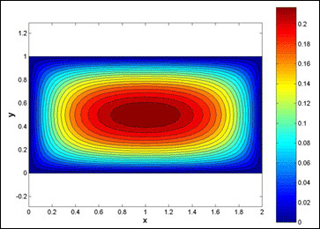MIT# [100% Off] NUMERICAL METHODS APPLIED TO CHaNUMERICAL METHODS APPLIED TO CHEMICAL ENGINEERINGEMICAL ENGINEERING Free Course Coupon

Updated: by ghatfanmerie

Click to get the course

8.0 hr
English
Free
Get the coupon in the end of description.

### Description

This course focuses on the use of modern computational and mathematical techniques in chemical engineering. Starting from a discussion of linear systems as the basic computational unit in scientific computing, methods for solving sets of nonlinear algebraic equations, ordinary differential equations, and differential-algebraic (DAE) systems are presented. Probability theory and its use in physical modeling is covered, as is the statistical analysis of data and parameter estimation. The finite difference and finite element techniques are presented for converting the partial differential equations obtained from transport phenomena to DAE systems. The use of these techniques will be demonstrated throughout the course in the MATLAB® computing environment.
If the coupon is not opening, disable Adblock, or try another browser.